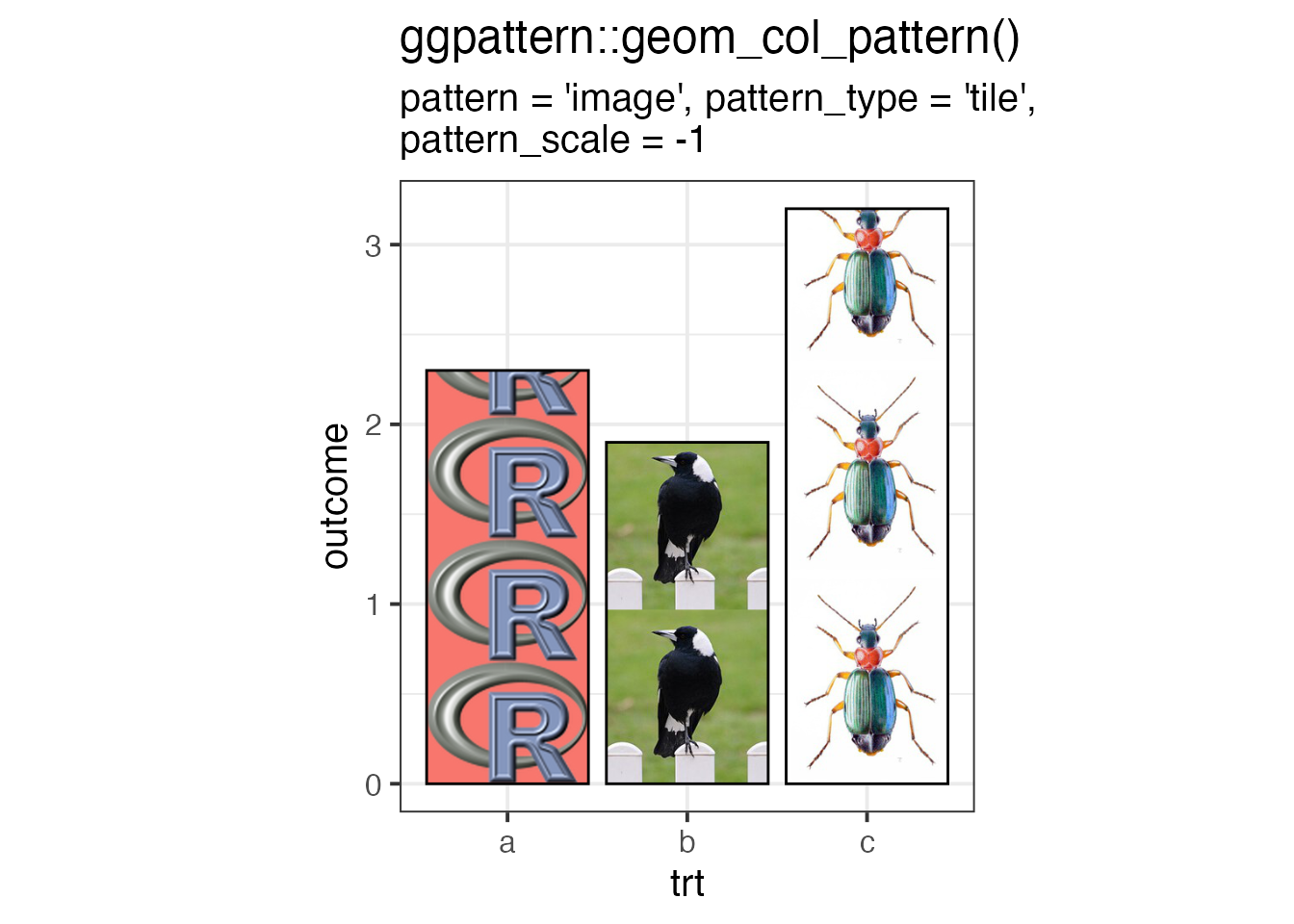suppressPackageStartupMessages({
library(dplyr)
library(ggplot2)
library(ggpattern)
})

## The image pattern - filling a geom with an image from file or URL

This pattern will load an image from a file or URL (pattern_filename) and display it within the geom.

Because the image will most likely not exactly cover the area of the geom, there are options to specify how the image is used to fill the region.

After expanding to cover the area, the image is then clipped to the boundary of the geom.

The files/URLs are loaded with magick::image_read which means that transparent images and SVGs are supported.

## image options

Aesthetic Description Default
pattern_filename Image filename or URL ’’
pattern_type Image scaling type ‘fit’
pattern_scale Extra scaling 1
pattern_gravity Position of image within area ‘center’
pattern_filter Filter to use when scaling ‘lanczos’
pattern_alpha Alpha NA
pattern_aspect_ratio Override aspect ratio NA
pattern_key_scale_factor Additional scale factor for legend 1
pattern_type Description pattern_scale pattern_gravity
squish distort the image to cover the bounding box of the region
fit scale the image such that either the width or the height of the image fits in the bounding box. Yes
expand scale the image beyond the bounding box and crop it such that the image fully covers the width and the height of the region
none position a single image in the region without attempting to scale to the bounding box size Yes Yes
tile repeat the image to cover the bounding box. Yes

Note: not all combinations of aesthetics are useful e.g. if pattern_type = 'fit' is specified, then pattern_scale has no effect.

## Example Data

These examples use some of the built-in images in the ggpattern package.

#~~~~~~~~~~~~~~~~~~~~~~~~~~~~~~~~~~~~~~~~~~~~~~~~~~~~~~~~~~~~~~~~~~~~~~~~~~~~~
# filenames of images
#~~~~~~~~~~~~~~~~~~~~~~~~~~~~~~~~~~~~~~~~~~~~~~~~~~~~~~~~~~~~~~~~~~~~~~~~~~~~~
logo_filename   <- system.file("img", "Rlogo.png" , package="png")
magpie_filename <- system.file("img", "magpie.jpg", package="ggpattern")
bug_filename    <- system.file("img", "bug.jpg"   , package="ggpattern")

seamless1 <- system.file("img", "seamless1.jpg"   , package="ggpattern")
seamless2 <- system.file("img", "seamless2.jpg"   , package="ggpattern")
seamless3 <- system.file("img", "seamless3.jpg"   , package="ggpattern")

#~~~~~~~~~~~~~~~~~~~~~~~~~~~~~~~~~~~~~~~~~~~~~~~~~~~~~~~~~~~~~~~~~~~~~~~~~~~~~
# Plotting data
#~~~~~~~~~~~~~~~~~~~~~~~~~~~~~~~~~~~~~~~~~~~~~~~~~~~~~~~~~~~~~~~~~~~~~~~~~~~~~
df1 <- data.frame(
trt      = c("a", "b", "c"),
outcome  = c(2.3, 1.9, 3.2),
gravity  = c('South', 'North', 'West'),
filltype = c('squish', 'fit' , 'expand'),
scale    = c(1, 2, 0.5),
filename = c(logo_filename, magpie_filename, bug_filename),
stringsAsFactors = FALSE
)
Example Data
trt outcome gravity filltype scale filename
a 2.3 South squish 1.0 /Library/Frameworks/R.framework/Versions/4.1/Resources/library/png/img/Rlogo.png
b 1.9 North fit 2.0 /private/var/folders/5p/78cv9fvn4xn_rbgxpx51q5n80000gn/T/Rtmp2nJhBJ/temp_libpath5905771c394/ggpattern/img/magpie.jpg
c 3.2 West expand 0.5 /private/var/folders/5p/78cv9fvn4xn_rbgxpx51q5n80000gn/T/Rtmp2nJhBJ/temp_libpath5905771c394/ggpattern/img/bug.jpg

## Example: pattern = 'image' - No Attempt at filling the area (pattern_type = 'none')

If pattern_type = 'none' then no attempt is made at snugly filling the area. Instead a single copy of the image is scaled (pattern_scale) and placed (pattern_gravity) in the geom area.

ggplot(df1, aes(trt, outcome)) +
geom_col_pattern(
aes(
fill            = trt,
pattern_gravity = I(gravity),
pattern_scale   = I(scale)
),
pattern          = 'image',
pattern_filename = logo_filename,
pattern_type     = 'none',
colour           = 'black'
) +
theme_bw(15) +
labs(
title    = "ggpattern::geom_col_pattern()",
subtitle = "pattern = 'image', pattern_type = 'none'"
) +
theme(legend.key.size = unit(1.5, 'cm')) +
coord_fixed(ratio = 1/2)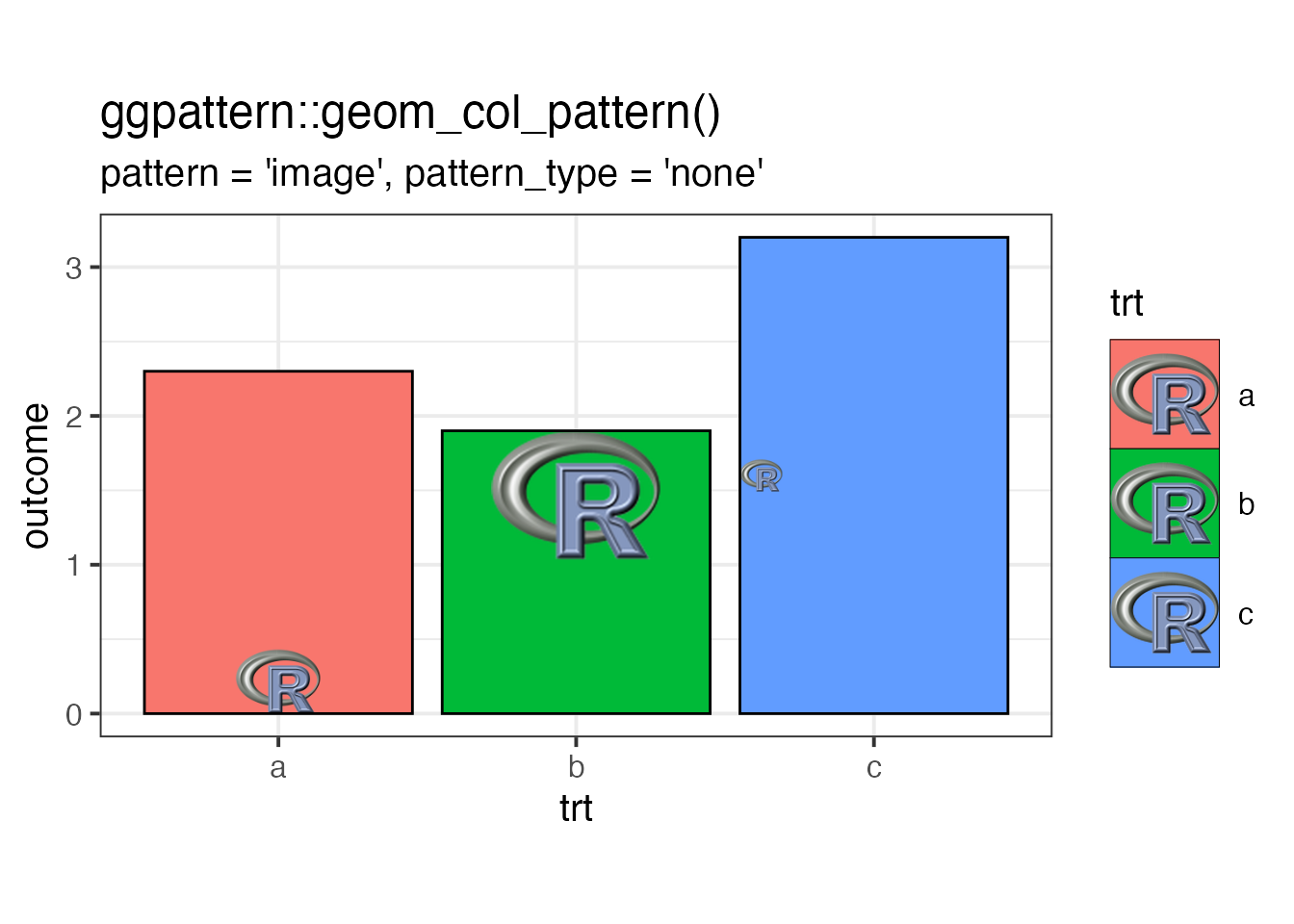## Example: pattern = 'image' - Covering the area (pattern_type = 'fit', 'squish', 'expand')

In the plot below, 3 different methods are used to cover the area of the geom. From left-to-right:

• pattern_type = 'squish' to force the image to cover the bounding box of the geom.
• pattern_type = 'fit' to force the image to cover the width (or height) yet still remain fully visible. pattern_gravity = 'North' floats the image to the top.
• pattern_type = 'expand scales the image (while preserving aspect ratio) such that the entire geom is covered.pattern_gravity = ‘West’ anchors the image to the left side of the geom.
ggplot(df1, aes(trt, outcome)) +
geom_col_pattern(
aes(
fill            = trt,
pattern_gravity = I(gravity),
pattern_type    = I(filltype)
),
pattern          = 'image',
colour           = 'black',
pattern_filename = logo_filename,

) +
theme_bw(15) +
labs(
title    = "ggpattern::geom_col_pattern()",
subtitle = "pattern = 'image'"
) +
# theme(legend.key.size = unit(1.5, 'cm')) +
theme(legend.position = 'none') +
coord_fixed(ratio = 1/2)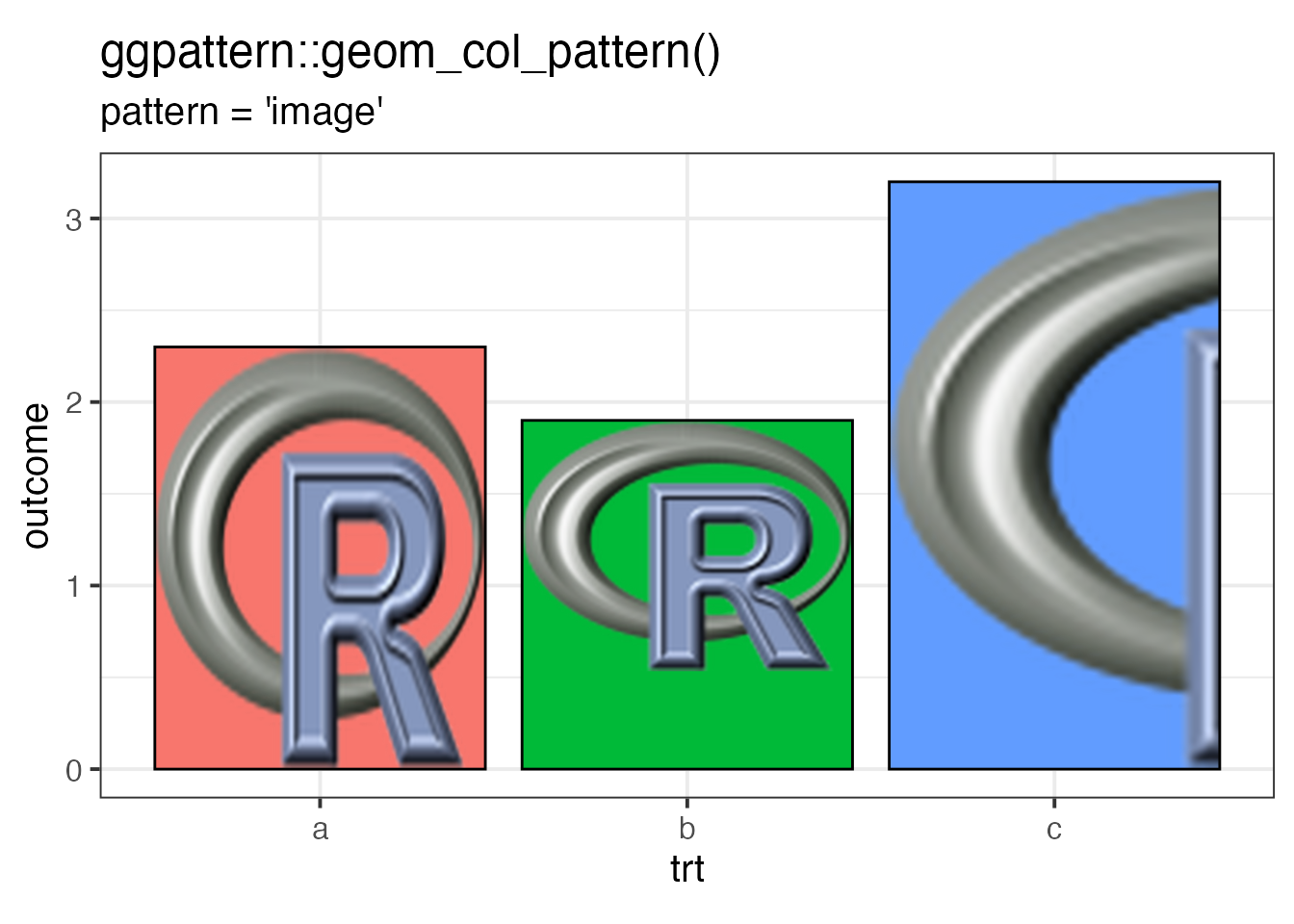## Example: pattern = 'image' - Tiling (pattern_type = 'tile')

When tiled, the image is replicated in order to cover the entire area of the geom. In this example, pattern_scale is also applied to the image so that it appears at different sizes.

ggplot(df1, aes(trt, outcome)) +
geom_col_pattern(
aes(
fill            = trt,
pattern_gravity = I(gravity),
pattern_scale   = I(scale)
),
pattern          = 'image',
pattern_type     = 'tile',
pattern_filename = logo_filename,
colour           = 'black'
) +
theme_bw(15) +
labs(
title    = "ggpattern::geom_col_pattern()",
subtitle = "pattern = 'image', pattern_type = 'tile'"
) +
# theme(legend.key.size = unit(1.5, 'cm')) +
theme(legend.position = 'none') +
coord_fixed(ratio = 1/2)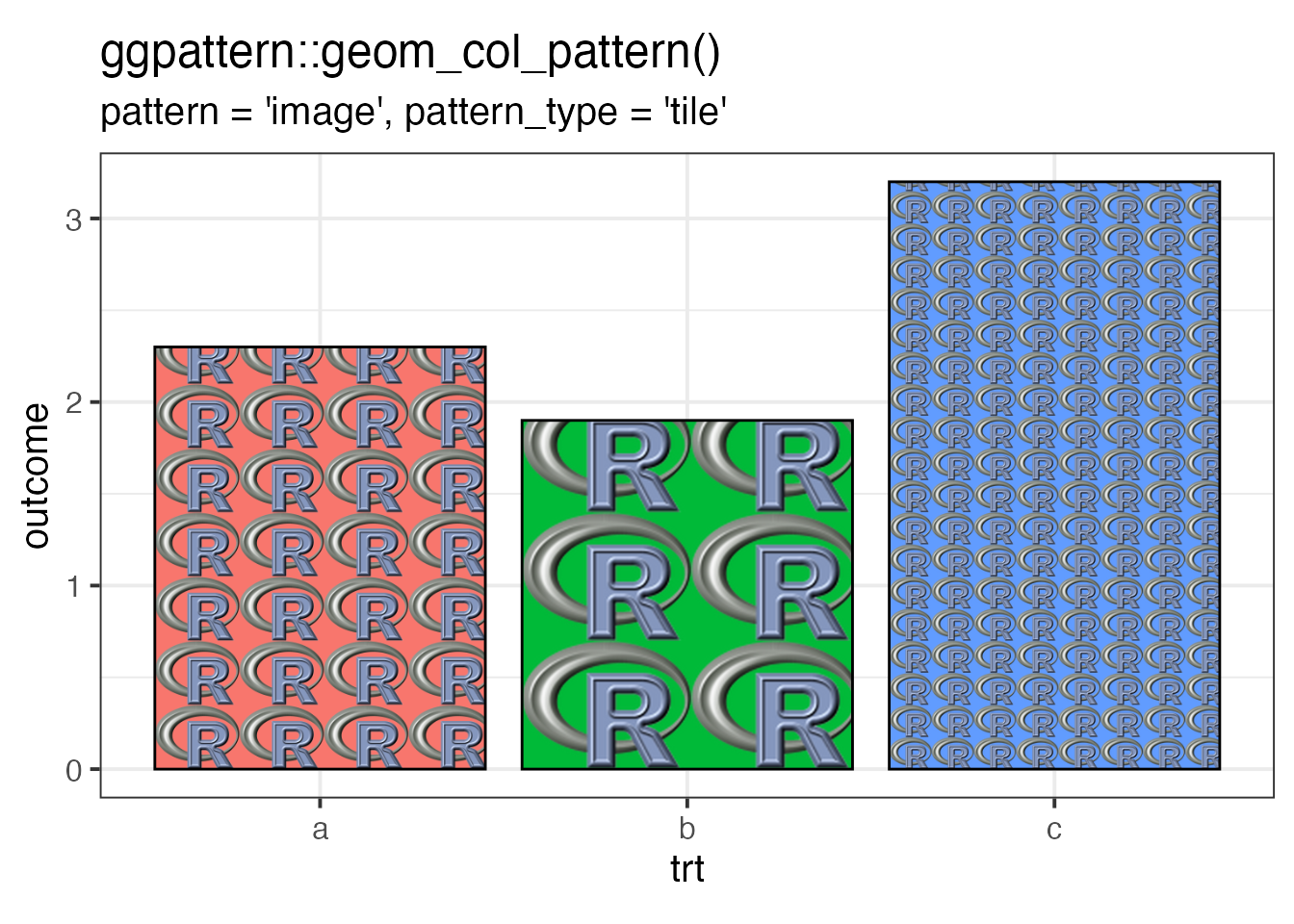## Example: pattern = 'image' - Tiling with seamless patterns

By choosing a seamlessly tiling image, then a tiled fill will not have visible discontinuities.

ggplot(mtcars) +
geom_density_pattern(
aes(
x = mpg,
pattern_filename = as.factor(cyl)
),
pattern      = 'image',
pattern_type = 'tile'
) +
theme_bw(15) +
theme(legend.key.size = unit(1.5, 'cm')) +
labs(
title    = "ggpattern::geom_density_pattern()",
subtitle = "pattern = 'image', pattern_type = 'tile'"
) +
scale_pattern_filename_manual(values = c(4 = seamless1, 6 = seamless2, 8 = seamless3)) +
coord_fixed(ratio = 80)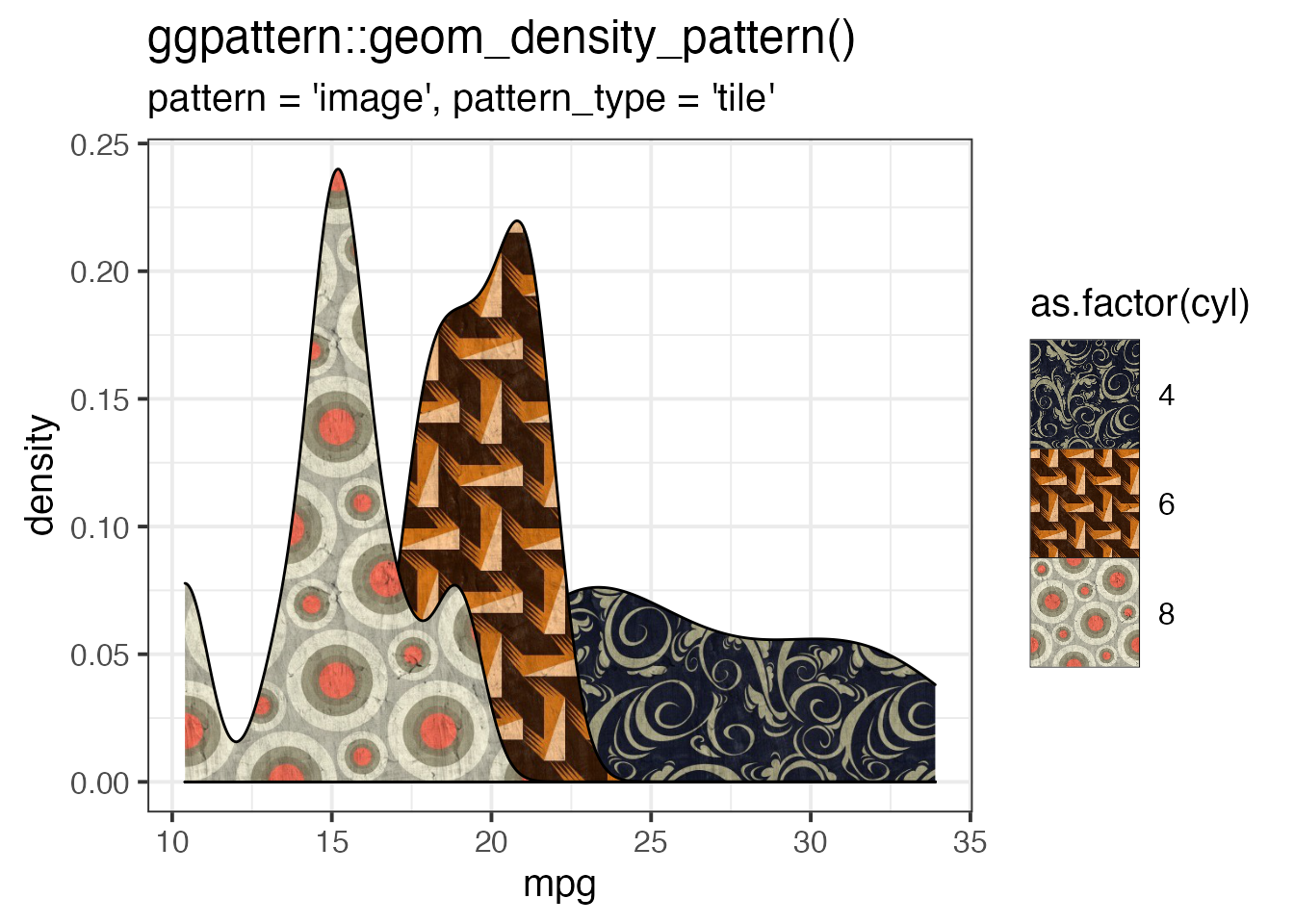## Example: pattern = 'image' - Tiling with seamless patterns (with scaling)

ggplot(mtcars) +
geom_density_pattern(
aes(
x = mpg,
pattern_filename = as.factor(cyl)
),
pattern       = 'image',
pattern_type  = 'tile',
pattern_scale = 0.5
) +
theme_bw(15) +
theme(legend.key.size = unit(2, 'cm')) +
labs(
title    = "ggpattern::geom_density_pattern()",
subtitle = "pattern = 'image', pattern_type = 'tile'"
) +
scale_pattern_filename_manual(values = c(4 = seamless1, 6 = seamless2, 8 = seamless3)) +
coord_fixed(ratio = 80)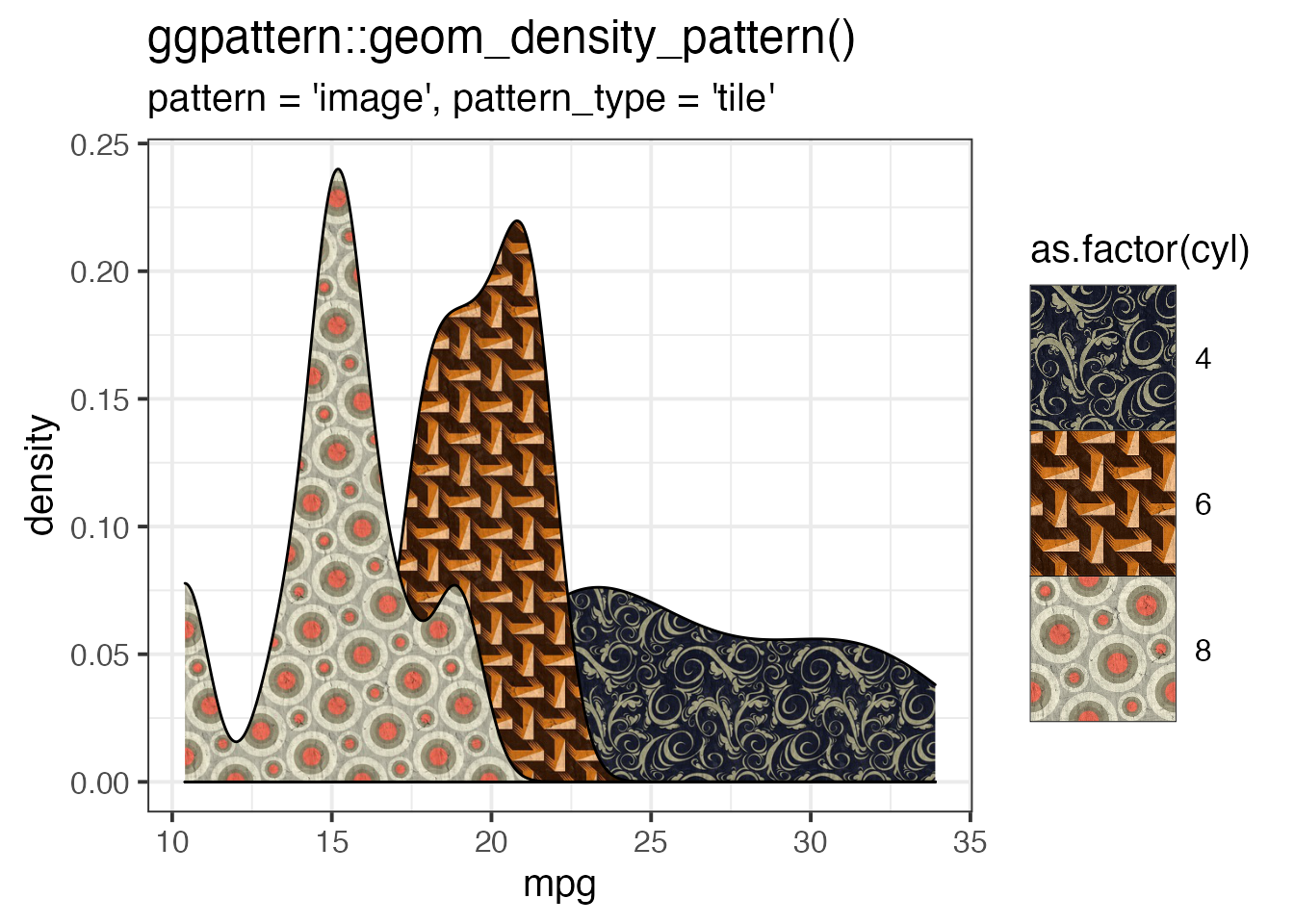## Example: pattern = 'image' - Tiling with fit to width (of element bounding box)

In a common case, the image to be tiled should be first scaled to the width of the bar to be tiled and then tiling appears like a vertical stacking. This is useful for bar charts.

Specify pattern_scale = -1 to fit the width of the geom, and pattern_scale = -2 to fit the height of the geom.

ggplot(df1, aes(trt, outcome)) +
geom_col_pattern(
aes(
fill             = trt,
pattern_filename = I(filename)
),
pattern          = 'image',
pattern_type     = 'tile',
pattern_scale    = -1,
colour           = 'black'

) +
theme_bw(15) +
labs(
title    = "ggpattern::geom_col_pattern()",
subtitle = "pattern = 'image', pattern_type = 'tile',\npattern_scale = -1"
) +
theme(legend.position = 'none') +
coord_fixed(ratio = 1)`